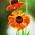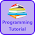# Programming Videos

## Search

### C Program to Count Number of Vowels and Consonants in a String

Write a C program to count number of vowels and consonants in a string. In this question, we have to write a code which takes an input string from a user and then print number of vowels and Consonants in a string.

C Program to check whether a input character is vowel or not

Programming Interview Questions

Before solving this problem let's understand what is vowel and consonant.

What is Vowel and Consonants ?

In english alphabet, A, E, I, O, U is called a vowel and remaining alphabets which is not a vowel is known as consonant.

How to read string with spaces using scanf

Programming Questions on Strings

C program to find the length of string without using strlen

## C Program to Count Number of Vowels and Consonants in a String

```#include<stdio.h>
#include<string.h>

int main(){

char str;

int i, vowel=0, consonant=0;

printf("Enter a string (Max 200 char)");
fgets(str,200,stdin);

/* Length of a string. */

int len = strlen(str);

/* Traverse a string */
for(i = 0; i < len; i++){

/* Check for vowel and increment the count. */

if( str[i]=='a' || str[i]=='A'
|| str[i]=='e' || str[i]=='E'
|| str[i]=='i' || str[i]=='I'
|| str[i]=='o' ||str[i]=='O'
|| str[i]=='u' ||str[i]=='U' ) {

vowel++;

/* Check for consonant. */

} else if((str[i]>='a' && str[i]<='z')
|| (str[i]>='A' && str[i]<='Z')) {

consonant++;
}
}

printf("Vowels = %d and Consonants = %d",vowel ,consonant);

return 0;
}

```

Output -

Enter a string  - I love cprogrammingcode.com

Vowels = 9 and Consonants = 15

1.#include
#include
using namespace std;
int main(){
char line;
int i,v,c,ch,d,s,o;
o=v=c=ch=d=s=0;
cout << "Enter a line of string: " << endl;
cin.getline(line, 150);
for(i=0;line[i]!='\0';++i)
{
if(line[i]=='a'
|| line[i]=='e'
|| line[i]=='i'
|| line[i]=='o'
|| line[i]=='u'
|| line[i]=='A'
|| line[i]=='E'
|| line[i]=='I'
|| line[i]=='O'
|| line[i]=='U')
++v;
else if((line[i]>='a'&& line[i]<='z')
|| (line[i]>='A'&& line[i]<='Z'))
++c;
else if(line[i]>='0'&&c<='9')
++d;
else if (line[i]==' ')
++s;
}
cout << " Vowels: " << v << endl;
cout << " Consonants: " << c << endl;
cout << " Digits: " << d << endl;
cout << " White Spaces: " << d << endl;
return 0;
}
Output

Enter a line of string:
programming is fun 1 234
Vowels: 5
Consonants: 11
Digits: 4
White Spaces: 4

1.